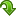ATOMS : Distfun details

# Distfun

Distribution functions
Details
Version
1.1
A more recent valid version with binaries for Scilab 6.0 exists: 1.1.1
Authors
Michael Baudin
Prateek Papriwal
Pierre Lecuyer
Luc Devroye
Jean-Philippe Chancelier
Michael A. Malcolm
Cleve B. Moler
George Marsaglia
Arif Zaman
Barry W. Brown
Owner Organization
INRIA, DIGITEO and others
Maintainers
Prateek Papriwal
Michael Baudin
Categories
Dependencies
Creation Date
December 13, 2016
Source created on
Scilab 6.0.x
Binaries available on
Scilab 6.0.x:Windows 64-bitLinux 64-bit
Install command
`--> atomsInstall("distfun")`
Report a bugDescription
```            The goal of this toolbox is to provide accurate distribution functions.
The provided functions are designed to be compatible with Matlab.

The goals of this toolbox are the following.
* All functions are tested with tables (actually, csv datasets).
The tests includes accuracy tests, so that the accuracy
should by from 13 to 15 significant digits in most cases.
* For each distribution, we have
* the probability distribution function (PDF)
* the cumulated distribution function (CDF)
* the inverse CDF
* the random number generator
* the statistics (mean and variance)
* The CDF provides the upper and the lower tail of the
distribution, for accuracy reasons.
* The uniform random numbers are of high quality.
The default is to use the Mersenne-Twister generator.
* Each function has a consistent help page.
This removes confusions in the meaning
of the parameters and clarifies the differences
with other computing languages (e.g. R).

The design is similar to Matlab's distribution functions.
A significant difference with Matlab's function is that both
the upper and lower tails are available in "distfun", while
Matlab only provides the lower tail.
Hence, "distfun" should provide a better accuracy when
probabilities close to 1 are computed (e.g. p=0.9999).

There are many interesting, positive, differences with Scilab, Stixbox, or other

http://forge.scilab.org/index.php/p/distfun/

Features
--------

For each distribution x, we provide five functions :
* distfun_xcdf : x CDF
* distfun_xinv : x Inverse CDF
* distfun_xpdf : x PDF
* distfun_xrnd : x random numbers
* distfun_xstat : x mean and variance

Distributions available :
* Beta (with x=beta)
* Binomial (with x=bino)
* Chi-Squared (with x=chi2)
* Extreme Value (with x=ev)
* Exponential (with x=exp)
* F (with x=f)
* Gamma (with x=gam)
* Geometric (with x=geo)
* Histogram (with x=histo)
* Hypergeometric (with x=hyge)
* Kolmogorov-Smirnov (with x=ks)
* LogNormal (with x=logn)
* LogUniform (with x=logu)
* Multinomial (with x=mn)
* Multivariate Normal (with x=mvn)
* Negative Binomial (with x=nbin)
* Noncentral F (with x=ncf)
* Noncentral T (with x=nct)
* Noncentral Chi-Squared (with x=ncx2)
* Normal (with x=norm)
* Poisson (with x=poi)
* T (with x=t)
* Truncated Normal (with x=tnorm)
* Uniform Discrete (with x=unid)
* Uniform (with x=unif)
* Weibull (with x=wbl)

Tutorial
* dispfun_tutorial : A tutorial of the Distfun toolbox.
* dispfun_plots : A collection of distribution function plots.

Support
* distfun_betainc : Regularized Incomplete Beta function
* distfun_erfcinv : Inverse erfc function
* distfun_gammainc : Regularized incomplete Gamma function
* distfun_genericpdf : Compute the PDF from the CDF.
* distfun_getpath : Returns path of current module
* distfun_histocreate : Creates an histogram
* distfun_inthisto : Discrete histogram
* distfun_permrnd : Random permutation
* distfun_plotintcdf :  Plots an integer CDF
* distfun_verboseset : Set verbose mode.

Weibull fitting
* distfun_wblfit : Weibull parameter estimates
* distfun_wblfitmm : Weibull parameter estimates with method of moments
* distfun_wbllike : Weibull negative log-likelihood
* distfun_wblplot : Weibull plot

Other fitting functions
* distfun_uniffitmm : Uniform parameter estimates with method of moments
* distfun_betafitmm : Beta parameter estimates with method of moments
* distfun_gamfitmm : Gamma parameter estimates with method of moments

Random Number Generator
* rng_overview : An overview of the Random Number Generators of the Distfun
toolbox.
* distfun_genget : Get the current random number generator
* distfun_genset : Set the current random number generator
* distfun_seedget : Get the current state of the current random number
generator
* distfun_seedset : Set the current state of the current random number
generator
* distfun_streamget : Get the current stream
* distfun_streaminit : Initializes the current stream
* distfun_streamset : Set the current stream

Multivariate vectors
* distfun_vectorrnd : Random vectors.
```Files (3)Source code archive
```
```Windows 64-bit binary for Scilab 6.0.x
```Windows version (x64)
Automatically generated by the ATOMS compilation chain

```Linux 64-bit binary for Scilab 6.0.x
```Linux version (x86_64)
Automatically generated by the ATOMS compilation chain

```News (0)Comments (0)Leave a comment
You must register and log in before leaving a comment.Email notifications
Send me email when this toolbox has changes, new files or a new release.